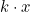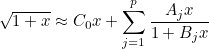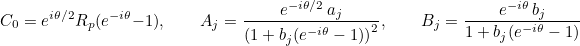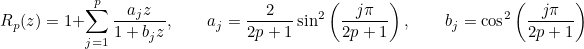# Special functions

In this page, the interface with special functions (such as Bessel functions) is detailed. This interface is achieved for the precision used by the user (double or multiple precision).

 GetKn returns Kn for a real argument GetJn returns Bessel function Jn for a real argument GetYn returns Neumann function Yn for a real argument GetDeriveJn returns Bessel function Jn and its derivative for a real argument ComputeBesselFunctions Computes Bessel functions for complex arguments ComputeDeriveBesselFunctions Computes Bessel functions and their derivatives for complex arguments ComputeBesselAndHankelFunctions Computes Bessel and Hankel functions of the first kind ComputeDeriveBesselAndHankel Computes Bessel and Hankel functions and their derivatives ComputeSphericalBessel Computes spherical Bessel functions ComputeDeriveSphericalBessel Computes spherical Bessel functions and their derivatives ComputeSphericalHankel Computes spherical Hankel functions ComputeDeriveSphericalHankel Computes spherical Hankel functions and their derivatives ComputeDeriveSphericalBesselHankel Computes spherical Hankel functions and Bessel functions and their derivatives ComputeDeriveRiccatiBessel Computes spherical Bessel-Riccati functions and their derivatives ComputeOrder Computes the maximal integer to use in spherical Bessel expansions ComputePowerI Computes the power of the imaginary number i GetLambertW0 Computes Lambert function GetWhittakerM Computes Whittaker function M for real arguments GetWhittakerW Computes Whittaker function W for real arguments GetMinimumDotProd returns the minimum of k \cdot x where x is contained in a rectangular bounding box GetMaximumDotProd returns the maximum of k \cdot x where x is contained in a rectangular bounding box CartesianToPolar conversion from cartesian coordinates to polar coordinates CartesianToSpherical conversion from cartesian coordinates to spherical coordinates SphericalToCartesian conversion from spherical coordinates to cartesian coordinates ComputePadeCoefficientsSqrt computes coefficients involved in Pade's expansion of square root

### GetKn

#### Syntax :

  Real_wp GetKn(int n, const Real_wp& x);


This function returns the modified Bessel function of the second kind denoted Kn for an integer n and a real argument x.

#### Example :

Real_wp y = GetKn(3, Real_wp(2.5));


#### Location :

BesselFunctionsInterface.cxx

### GetJn

#### Syntax :

  Real_wp GetJn(int n, const Real_wp& x);


This function returns the Bessel function Jn for an integer n and a real argument x.

#### Example :

Real_wp y = GetJn(3, Real_wp(2.5));


#### Location :

BesselFunctionsInterface.cxx

### GetYn

#### Syntax :

  Real_wp GetYn(int n, const Real_wp& x);


This function returns the Neumann function Yn (Bessel function of the second kind) for an integer n and a real argument x.

#### Example :

Real_wp y = GetYn(3, Real_wp(2.5));


#### Location :

BesselFunctionsInterface.cxx

### GetDeriveJn

#### Syntax :

  void GetDeriveJn(int n, const Real_wp& x, Real_wp& Jn, Real_wp& dJn);


This function computes the Bessel function Jn and its derivative for an integer n and a real argument x.

#### Example :

Real_wp Jn, dJn;
// evaluating J_3 and J'_3 for x = 2.5
GetDeriveJn(3, Real_wp(2.5), Jn, dJn);


#### Location :

BesselFunctionsInterface.cxx

### ComputeBesselFunctions

#### Syntax :

  void ComputeBesselFunctions(int n0, int n, const Real_wp& x, VectReal_wp& Jn);
void ComputeBesselFunctions(int n0, int n, const Complex_wp& z, VectComplex_wp& Jn);
void ComputeBesselFunctions(int n0, int n, const Complex_wp& z, VectComplex_wp& Jn, VectComplex_wp& Yn);


This function computes the Bessel functions Jk for k=n0 until k=n0+n-1 such that n Bessel functions are computed. In the last syntax, Bessel functions of the first and second kind are both computed. It is more efficient to compute a sequence of Bessel functions with this function (since it uses the recurrence relation) than evaluating separately each Bessel function with GetJn/GetYn.

#### Example :

// for a real argument
VectReal_wp Jn;
// we want to evaluate J_2, J_3, ..., J_12 at x = 2.5
// n0 = 2 and n = 11
ComputeBesselFunctions(2, 11, Real_wp(2.5), Jn);

// for a complex argument z = 2.2+0.3i
VectComplex_wp Jn_c;
ComputeBesselFunctions(2, 11, Complex_wp(2.2, 0.3), Jn_c);

// for a complex argument, both Jn and Yn can be computed
VectComplex_wp Yn_c;
ComputeBesselFunctions(2, 11, Complex_wp(2.2, 0.3), Jn_c, Yn_c);


#### Location :

BesselFunctionsInterface.cxx

### ComputeDeriveBesselFunctions

#### Syntax :

  void ComputeDeriveBesselFunctions(int n0, int n, const Real_wp& x, VectReal_wp& Jn, VectReal_wp& dJn);


This function computes the Bessel functions Jk for k=n0 until k=n0+n-1 and its derivatives such that n Bessel functions are computed. It is more efficient to compute a sequence of Bessel functions with this function (since it uses the recurrence relation) than evaluating separately each Bessel function with GetDeriveJn.

#### Example :

// for a real argument
VectReal_wp Jn, dJn;
// we want to evaluate J_2, J_3, ..., J_12 at x = 2.5
// and derivatives J'_2, J'_3, ..., J'_12 at the same point
// n0 = 2 and n = 11
ComputeDeriveBesselFunctions(2, 11, Real_wp(2.5), Jn, dJn);


#### Location :

BesselFunctionsInterface.cxx

### ComputeBesselAndHankelFunctions

#### Syntax :

  void ComputeBesselAndHankelFunctions(const Real_wp& n0, int n, const Complex_wp& z, VectComplex_wp& Jn, VectComplex_wp& Hn);


This function computes the Bessel functions Jk for k=n0 until k=n0+n-1 and Hankel functions such that n Bessel and Hankel functions are computed. The beginning order n0 is real (and not only integer) and the argument z is complex.

#### Example :

// for a complex argument
VectComplex_wp Jn, Hn;
// we want to evaluate J_{2.5}, J_{3.5}, ..., J_{12.5} at z = 2.2+0.3i
// and Hankel functions H_{2.5}, H_{3.5}, ..., H_{12.5} at the same point
// n0 = 2.5 and n = 11
ComputeBesselAndHankelFunctions(Real_wp(2.5), 11, Complex_wp(2.2, 0.3), Jn, Hn);


#### Location :

BesselFunctionsInterface.cxx

### ComputeDeriveBesselAndHankel

#### Syntax :

   void ComputeDeriveBesselAndHankel(const Real_wp& n0, int n, const Complex_wp& z,
VectComplex_wp& Jn, VectComplex_wp& Hn, VectComplex_wp& dJn, VectComplex_wp& dHn);


This function computes the Bessel functions Jk for k=n0 until k=n0+n-1 and its derivatives such that n Bessel and Hankel functions are computed with their derivatives. The beginning order n0 is real (and not only integer) and the argument z is complex.

#### Example :

// for a complex argument
VectComplex_wp Jn, Hn, dJn, dHn;
// we want to evaluate J_{2.5}, J_{3.5}, ..., J_{12.5} at z = 2.2+0.3i
// and Hankel functions H_{2.5}, H_{3.5}, ..., H_{12.5} at the same point
// and all their derivatives that will be stored in dJn and dHn
// n0 = 2.5 and n = 11
ComputeDeriveBesselAndHankel(Real_wp(2.5), 11, Complex_wp(2.2, 0.3), Jn, Hn, dJn, dHn);


#### Location :

BesselFunctionsInterface.cxx

### ComputeSphericalBessel

#### Syntax :

  void ComputeSphericalBessel(int n, const Real_wp& x, VectReal_wp& Jn);


This function computes the n first spherical Bessel functions jk (k goes from 0 until n-1) for a real argument x.

#### Example :

// for a real argument
VectReal_wp Jn;
// we want to evaluate spherical Bessel functions j_0, j_1, ..., j_{10} at x=2.5
ComputeSphericalBessel(11, Real_wp(2.5), Jn);


#### Location :

BesselFunctionsInterface.cxx

### ComputeDeriveSphericalBessel

#### Syntax :

  void ComputeDeriveSphericalBessel(int n, const Real_wp& x, VectReal_wp& Jn, VectReal_wp& dJn);


This function computes the n first spherical Bessel functions jk (k goes from 0 until n-1) and their derivatives for a real argument x.

#### Example :

// for a real argument
VectReal_wp Jn, dJn;
// we want to evaluate spherical Bessel functions j_0, j_1, ..., j_{10} at x=2.5
// and derivatives j'_0, j'_1, ..., j'_{10} at the same point
ComputeDeriveSphericalBessel(11, Real_wp(2.5), Jn, dJn);


#### Location :

BesselFunctionsInterface.cxx

### ComputeSphericalHankel

#### Syntax :

  void ComputeSphericalHankel(int n, const Real_wp& x, VectComplex_wp& Hn);


This function computes the n first spherical Hankel functions of the first kind hk (k goes from 0 until n-1) for a real argument x.

#### Example :

// for a real argument
VectComplex_wp Hn;
// we want to evaluate spherical Hankel functions h_0, h_1, ..., h_{10} at x=2.5
ComputeSphericalHankel(11, Real_wp(2.5), Hn);


#### Location :

BesselFunctionsInterface.cxx

### ComputeDeriveSphericalHankel

#### Syntax :

  void ComputeDeriveSphericalHankel(int n, const Real_wp& x, VectComplex_wp& Hn, VectComplex_wp& dHn);


This function computes the n first spherical Hankel functions of the first kind hk (k goes from 0 until n-1) and their derivatives for a real argument x.

#### Example :

// for a real argument
VectComplex_wp Hn, dHn;
// we want to evaluate spherical Hankel functions h_0, h_1, ..., h_{10} at x=2.5
// and derivatives h'_0, h'_1, ..., h'_{10} at the same point
ComputeDeriveSphericalHankel(11, Real_wp(2.5), Hn, dHn);


#### Location :

BesselFunctionsInterface.cxx

### ComputeDeriveSphericalBesselHankel

#### Syntax :

   void ComputeDeriveSphericalBesselHankel(const Real_wp& n0, int n, const Complex_wp& z, VectComplex_wp& Jn, VectComplex_wp& Hn,
VectComplex_wp& dJn, VectComplex_wp& dHn);


This function computes the spherical Bessel and Hankel functions of the first kind jk, hk (k goes from n0-1/2 until n0+n-3/2) and their derivatives for a complex argument z. n0 should be set to 0.5 in order to obtain spherical Bessel functions with integers.

#### Example :

// spherical Bessel and Hankel functions are stored in arrays
VectComplex_wp Jn, dJn, Hn, dHn;
// we want to evaluate spherical Bessel functions j_0, j_1, ..., j_{10} at z=2.2+0.3i
// and derivatives j'_0, j'_1, ..., j'_{10} at the same point
// and also spherical Hankel functions of the first kind
ComputeDeriveSphericalBesselHankel(Real_wp(0.5), 11, Complex_wp(2.2,0.3), Jn, Hn, dJn, dHn);


#### Location :

BesselFunctionsInterface.cxx

### ComputeDeriveRiccatiBessel

#### Syntax :

   void ComputeDeriveRiccatiBessel(const Real_wp& n0, int n, const  Complex_wp& z, VectComplex_wp& Jn, VectComplex_wp& Hn,
VectComplex_wp& dJn, VectComplex_wp& dHn, VectComplex_wp& Psi_n, VectComplex_wp& Xi_n,
VectComplex_wp& dPsi_n, VectComplex_wp& dXi_n );


This function computes the spherical Bessel and Hankel functions of the first kind jk, hk (k goes from n0-1/2 until n0+n-3/2) and their derivatives for a complex argument z. It computes also Riccati-Bessel functions Sn and ξn (associated respectively with spherical Bessel functions and Hankel functions). n0 should be set to 0.5 in order to obtain spherical Bessel functions with integers.

#### Example :

// spherical Bessel and Hankel functions are stored in arrays
VectComplex_wp Jn, dJn, Hn, dHn, Sn, dSn, Xi_n, dXi_n;
// we want to evaluate spherical Bessel functions j_0, j_1, ..., j_{10} at z=2.2+0.3i
// and derivatives j'_0, j'_1, ..., j'_{10} at the same point
// and also spherical Hankel functions of the first kind
// and Riccati-Bessel functions S_0, S_1, ..., Xi_0, Xi_1, ...
ComputeDeriveRiccatiBessel(Real_wp(0.5), 11, Complex_wp(2.2,0.3), Jn, Hn, dJn, dHn, Sn, Xi_n, dSn, dXi_n);


#### Location :

BesselFunctionsInterface.cxx

### ComputeOrder

#### Syntax :

  int ComputeOrder(const Real_wp& ka, const Real_wp& epsilon)


This function returns the number of spherical Bessel functions to take into account for the scattering of a sphere a radius a and wave number k (ka is the product of k and a) to obtain an accuracy of epsilon.

#### Example :

// for k = 2 pi, and a = 5, ka = 10 pi
// number of spherical Bessel functions for machine precision accuracy ?
int n = ComputeOrder(10*pi_wp, epsilon_machine);


#### Location :

BesselFunctionsInterface.cxx

### ComputePowerI

#### Syntax :

  Complex_wp ComputePowerI(int n)


This function returns in where i the pure imaginary number.

#### Example :

// z = i^n
Complex_wp z = ComputePowerI(n);


#### Location :

BesselFunctionsInterface.cxx

### GetLambertW0

#### Syntax :

  Real_wp GetLambertW0(const Real_wp& x)


This function returns the Lambert function W0 for a real argument x.

#### Example :

Real_wp w = GetLambertW0(Real_wp(2.4));


#### Location :

BesselFunctionsInterface.cxx

### GetWhittakerM, GetWhittakerW

#### Syntax :

   Real_wp GetWhittakerM(const Real_wp& Kappa, const Real_wp& mu, const Real_wp& x)
Real_wp GetWhittakerW(const Real_wp& Kappa, const Real_wp& mu, const Real_wp& x)


The function GetWhittakerM returns the Whittaker function Mκ, μ(x) where κ, μ and x are real. The function GetWhittakerW returns Wκ, μ(x).

#### Example :

Real_wp kappa(0.8), mu(1.2), x(2.5);
Real_wp m = GetWhittakerM(kappa, mu, x);
Real_wp w = GetWhittakerW(kappa, mu, x);


#### Location :

BesselFunctionsInterface.cxx

### GetMinimumDotProd

#### Syntax :

   Real_wp GetMinimumDotProd(TinyVector k, Real_wp xmin, Real_wp xmax, Real_wp ymin, Real_wp ymax, Real_wp zmin, Real_wp zmax);
Real_wp GetMaximumDotProd(TinyVector k, Real_wp xmin, Real_wp xmax, Real_wp ymin, Real_wp ymax, Real_wp zmin, Real_wp zmax);


The function GetMinimumDotProd returns the minimal value ofwhere x is contained in the box [xmin, xmax] x [ymin, ymax] x[zmin, zmax]. The function GetMaximumDotProd returns the maximal value. These two functions are implemented for 2-D and 3-D vectors.

#### Example :

R2 k(-0.4, 0.9);
Real_wp a = -2.0, b = 3.0, c = -1.0, d = 4.0;

// minimum of k . x where x is in the square [a, b] x [c, d]
// the two last arguments (zmin, zmax) are used in 3-D only
Real_wp s = GetMinimumDotProd(k, a, b, c, d, c, d);


#### Location :

Share/CommonMontjoie.cxx

### CartesianToPolar

#### Syntax :

  void CartesianToPolar(Real_wp x, Real_wp y, Real_wp& r, Real_wp& theta);


This function computes the polar coordinates (r, theta) from cartesian coordinates (x, y).

#### Example :

// cartesian coordinates
Real_wp x = 0.4, y = 0.8;

// conversion to polar coordinates
Real_wp r, theta;
CartesianToPolar(x, y, r, theta);


#### Location :

Share/CommonMontjoie.cxx

### CartesianToSpherical

#### Syntax :

  void CartesianToSpherical(Real_wp x, Real_wp y, Real_wp z, Real_wp& r, Real_wp& theta, Real_wp& phi);
void CartesianToSpherical(Real_wp x, Real_wp y, Real_wp z, Real_wp& r, Real_wp& theta, Real_wp& phi, Real_wp& cos_teta, Real_wp& sin_teta);


This function computes the spherical coordinates (r, theta, phi) from cartesian coordinates (x, y, z). In the second syntax, you can also retrieve the cosine and sine of theta.

#### Example :

// cartesian coordinates in 3-D
Real_wp x = 0.4, y = 0.8, z = -2.1;

// conversion to spherical coordinates
Real_wp r, theta, phi;
CartesianToSpherical(x, y, z,  r, theta, phi);


#### Location :

Share/CommonMontjoie.cxx

### SphericalToCartesian

#### Syntax :

  void SphericalToCartesian(Real_wp r, Real_wp theta, Real_wp phi, Real_wp& x, Real_wp& y, Real_wp& z);


This function computes the cartesian coordinates (x, y, z) from spherical coordinates (r, theta, phi).

#### Example :

// spherical coordinates of a 3-D point
Real_wp r = 0.4, theta = pi_wp/4, phi = pi_wp / 3;

// conversion to cartesian coordinates
Real_wp x, y, z;
SphericalToCartesian(r, theta, phi, x, y, z);


#### Location :

Share/CommonMontjoie.cxx

#### Syntax :

  void ComputePadeCoefficientsSqrt(Real_wp theta, int p, Complex_wp& C0, VectComplex_wp& Aj, VectComplex_wp& Bj);


This function computes the complex Pade approximant of the square root:whereThe angle θ and the integer p are given as input arguments of the function. Coefficients C0, Aj, Bj are output arguments.

#### Example :

Complex_wp C0;
VectComplex_wp Aj, Bj;

// coefficients for theta = pi/4 and p = 10